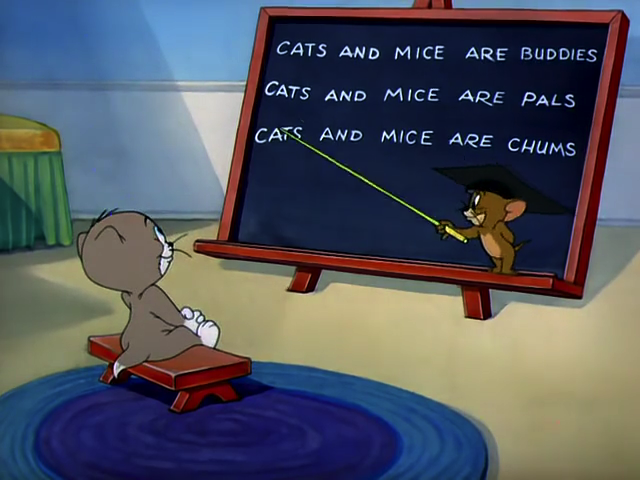# 17. Dr. Mouse and Elo Rating

Elo Rating，即 ELO 等级分制度，是由匈牙利裔美国物理学家 Arpad Elo 创建的一个衡量各类对弈活动水平的评价方法，是当今对弈水平评估的公认的权威方法。Elo Rating 的计算方法很多，其中一种比较公认的算法是这样的：

$$P_{A}={\frac 1{1+10^{{(R_{B}-R_{A})/400}}}},$$

$$P_{B}={\frac 1{1+10^{{(R_{A}-R_{B})/400}}}}.$$

$$E_A=P_A, E_B=P_B,$$

$$R_{A}^{\prime }=R_{A}+K_A \times (S_{A}-E_{A}).$$

$$R_{B}^{\prime }=R_{B}+K_B \times (S_{B}-E_{B}).$$

$$K_X = \begin{cases} 32 & {R_X < 2100}\ 24 & {2100 \leq R_X < 2400}\ 16 & {R_X \geq 2400} \end{cases}$$Jerry 在 Tom 不在的时候给小猫代课。比如说今天就讲授了 Elo Rating 的计算方法。但由于讲课水平太迷，小猫完全不明白，在做作业的时候也遇到的很大的困难。你的任务就是帮小猫完成作业（作业要求见输入格式）。

### 输入格式

• 1 X Y 表示发生了一场有胜负的对决。X 和 Y 为用户的编号。X 胜，Y 负，保证 X 和 Y 均合法。
• 2 X Y 与询问 1 相同，但表示发生了一场平局。
• 3 X 查询 X 用户当前的等级分，保证 X 合法。

### 样例

Input
7
1 1 2
1 1 3
2 2 3
3 1
3 2
1 2 3
3 3

Output
1531.263693
1484.033908
1468.671614


50 人解决，53 人已尝试。

81 份提交通过，共有 147 份提交。

2.9 EMB 奖励。